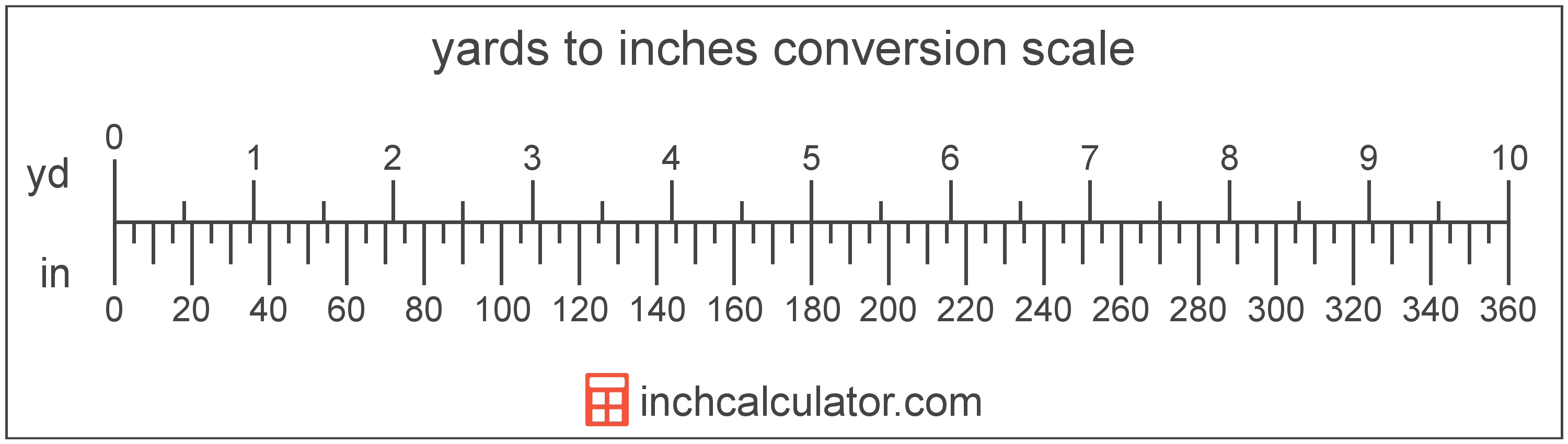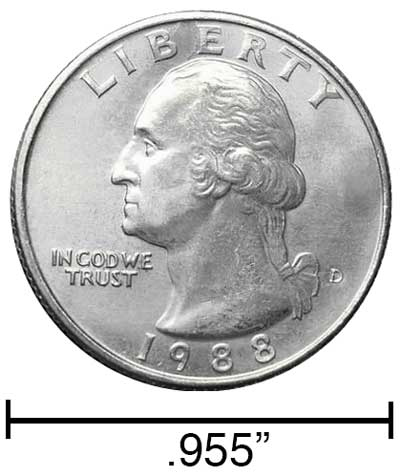Convert Inches to Yards

Enter the length in inches below to get the value converted to yards.

Results in Yards:1 in = 0.027778 yd
Do you want to convert yards to inches?

How to Convert Inches to YardsTo convert an inch measurement to a yard measurement, multiply the length by the conversion ratio. One inch is equal to 0.027778 yards, so use this simple formula to convert:

yards = inches × 0.027778

The length in yards is equal to the inches multiplied by 0.027778.

For example, here's how to convert 5 inches to yards using the formula above.
5" = (5 × 0.027778) = 0.138889 yd

Our inch fraction calculator can add inches and yards together and it also automatically converts the results to US customary, imperial and SI metric values.

Inches and yards are both units used to measure length. Keep reading to learn more about each unit of measure.

Inches

An inch is a unit of linear length measure equal to 1/12 of a foot or 1/36 of a yard. Because the international yard is legally defined to be equal to exactly 0.9144 meters, one inch is equal to 2.54 centimeters.

The inch is a US customary and imperial unit of length. Inches can be abbreviated as in, for example 1 inch can be written as 1 in.

Inches can also be denoted using the symbol, otherwise know as a double-prime. Often a double-quote (") is used instead of a double-prime for convenience. Using the double-prime, 1 in can be written as 1″.The standard ruler has 12", and are a common measuring tool for measuring inches. They are also often measured using tape measures which commonly come in lengths from 6' - 35'. Other types of measuring devices include scales, calipers, measuring wheels, micrometers, yardsticks, and even lasers.

Yards

The yard is a unit of length measurement equal to 3 feet or 36 inches. The international yard is legally defined to be equal to exactly 0.9144 meters.

The yard is a US customary and imperial unit of length. Yards can be abbreviated as yd, for example 1 yard can be written as 1 yd.

We recommend using a ruler or tape measure for measuring length, which can be found at a local retailer or home center. Rulers are available in imperial, metric, or combination with both values, so make sure you get the correct type for your needs.

If you need a ruler you can also download and print our free printable rulers, which include both imperial and metric measurements.

Inch Measurements and Equivalent Yard Conversions

Common inch values converted to the equivalent yard value
Inches Yards
1" 0.027778 yd
2" 0.055556 yd
3" 0.083333 yd
4" 0.111111 yd
5" 0.138889 yd
6" 0.166667 yd
7" 0.194444 yd
8" 0.222222 yd
9" 0.25 yd
10" 0.277778 yd
11" 0.305556 yd
12" 0.333333 yd
13" 0.361111 yd
14" 0.388889 yd
15" 0.416667 yd
16" 0.444444 yd
17" 0.472222 yd
18" 0.5 yd
19" 0.527778 yd
20" 0.555556 yd
21" 0.583333 yd
22" 0.611111 yd
23" 0.638889 yd
24" 0.666667 yd
25" 0.694444 yd
26" 0.722222 yd
27" 0.75 yd
28" 0.777778 yd
29" 0.805556 yd
30" 0.833333 yd
31" 0.861111 yd
32" 0.888889 yd
33" 0.916667 yd
34" 0.944444 yd
35" 0.972222 yd
36" 1 yd
37" 1.0278 yd
38" 1.0556 yd
39" 1.0833 yd
40" 1.1111 yd

References

1. National Institute of Standards and Technology, Handbook 133 - 2019 Edition, https://www.nist.gov/sites/default/files/documents/2017/04/28/12-apde-gentab-11-hb133-final.pdf

More Inch Length Conversions

US Customary & Imperial Units
Convert to Miles
1" is equal to 1.5783E-5 miles
Convert to Feet
1" is equal to 0.083333 feet
SI Units
Convert to Kilometers
1" is equal to 2.54E-5 kilometers
Convert to Meters
1" is equal to 0.0254 meters
Convert to Centimeters
1" is equal to 2.54 centimeters
Convert to Millimeters
1" is equal to 25.4 millimeters
Convert to Micrometers
1" is equal to 25,400 micrometers
Convert to Nanometers
1" is equal to 25,400,000 nanometers